People love water in video games. I can’t count the number of times I’ve heard people talking about how realistic the water is in the latest and greatest game. I make no claims to be immune to it either as I’ve stopped to appreciate it’s beauty in many a game. :)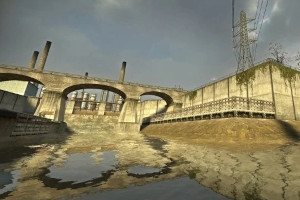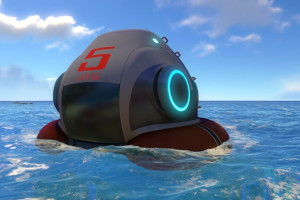Water in Half Life 2 and Subnautica.

Rendering and animating water are both pretty big topics, so this article is going to detail an algorithm for procedurally simulating interactive water waves using FFTs. Something like this!

Keep in mind that it’s a just surface simulation, so horizontal mouse movements faster than the wave speed won’t be very satisfying. ;) The algorithm itself extends to 3D easily enough, though I’ll be doing it in 2D so it’s easier to make quick visualizations.

## Lots of Water Algorithms

There’s actually quite a lot of algorithms for simulating water. One of the simplest is to treat the water surface like a grid. For each cell you store the height and vertical velocity so you can simulate the motion. To step time forward you move the height with the velocity, then feed the height back into the velocity (when the water level is high it wants to accelerate back down, and vice versa). This makes the water bob up and down. To make the waves propagate you just need to average each cell with it’s neighbors. This easy filtering method is pretty effective and very fast! To interact with the water, you just change the height or velocity values. Though delightfully simple to implement, it does have it’s issues. For one, it’s difficult to make framerate independent as the waves will move the same amount each step. It also doesn’t really move like water waves for a few reasons.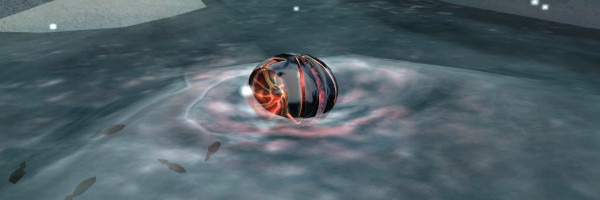Interactive water ripples in Metroid Prime

This is where fourier based methods come in using Fast Fourier Transforms, or FFTs, to simulate water. It gives you an intuitive way to perform much fancier filtering, and without a lot of extra cost! Instead of treating the grid like a bunch of locations that have waves in them somehow, the fourier transform lets you convert back and forth between a grid and waves. It’s much easier to handle some of water’s unique characteristics when dealing with waves. For example, unlike sound or light, water waves don’t all move at the same speed. Long waves move faster than little waves giving water it’s unique pulsing look as the different waves interact in complicated ways. Jump Trajectory has a nice overview video about how they implemented the FFT based animation in Unity. It’s an excellent video, but it’s light on the details and assumes you already know how FFTs work. It also doesn’t implement a simulation. That’s what I’d like to fill in with this article.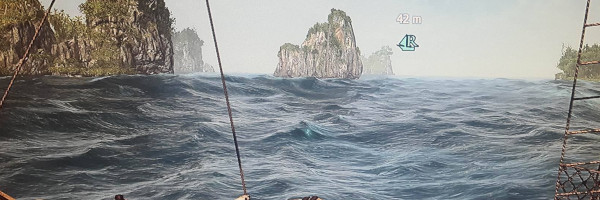FFT based ocean waves in Assasin’s Creed

At the extreme end of the spectrum, you can simulate the whole volume of water using fluid dynamics instead of just approximating it’s surface. Waves can crest and fall over, they can splash, and the water can even pour across surfaces. Unsurprisingly, adding another dimension is really expensive and simulating the whole volume usually isn’t feasible except at low resolutions. While I’ve never implemented proper fluid dynamics myself, it really doesn’t look all that hard. Ten Minute Physics recently posted a video on implementing the FLIP algorithm in 2D. It looks surprisingly simple and fun! There’s also a neat 2D fluid simulation library based on Box2D called Liquid Fun.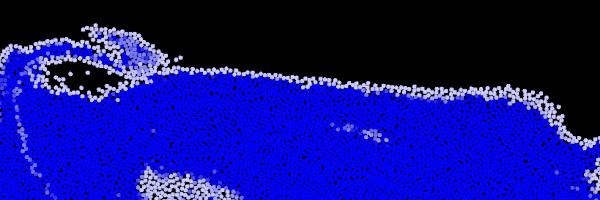A fluid simulation using the FLIP algorithm from the 10 Minute Physics blog

## A Quick Water Wave Primer

The first thing to know about waves (or almost any periodic motion really) is that it’s just energy that’s stuck in a loop. In the case of water waves, the energy bounces back between kinetic and potential energy. When the water is high, gravity pulls it down. It’s momentum causes it to overshoot the equilibrium point, and it move down too far. Then the pressure of the water around it increases and pushes it back up. Then it overshoots again, and goes too high. Rinse and repeat endlessly. (pun intended)A phase plot of the water surface

# The Simplest Wave

Let’s start with a simple wave model: a sine wave. (I swear there will be very little trigonometry involved in this article.) You’ll probably remember that `y = sin(x)` gives you a nice wobbly line. If you want to animate it, you just need to change the phase using time: `y = sin(x - time)`. That produces a nice little animated wave that moves left to right like this one.

A simple animated wave

If the blue line is the water height, can you guess what the red line is? (Hint: watch the red arrow.) It’s the water’s vertical velocity. When the velocity is up it makes the height move up, and when the height is up it makes the velocity move down just like in the phase plot. Another way of looking at it is that the velocity wave pulls the height wave. If you swapped their positions they would pull wave to the left instead. The velocity will be important later when we talk about simulating waves, but for now let’s take a shortcut and just animate the height.

# A Better Wave

Does the animated sine wave look like a water wave? Well… not really. First of all, if you compare it to the ocean waves in the Assasin’s Creed screenshot above, it’s the wrong shape. Those waves have pointy peaks and wide troughs. The reason for this is because real water waves don’t just bob up and down, they roll in roughly circlular path. This is called a trochoidal wave (sometimes called gerstner waves in computer graphics stuff). That’s easy enough! Instead of just moving the vertexes up and down, we add something to move them back and forth too. While we are making changes, lets make add a wavelength and amplitude property. Now our height is:

`y = amplitude*sin(x/wavelength - time)`

(Technically you need π represented in there for the wavelength, but if you don’t care about using real units, then it doesn’t matter.)

Each time a wave finishes a cycle, it moves forward by one wavelength. This is a mild problem because longer waves will move faster. If we want our waves to move at the same speed, then we need to slow down waves proportionally by their wavelength. Easy enough, just divide time to slow it down:

`y = amplitude*sin(x/wavelength - time/wavelength)`

Also for reasons we’ll get into later when we talk about FFTs and complex numbers, let’s swap that for a cosine instead. To make the trochoidal shape, we just need a matched pair of sines and cosines. The final wave code would look something like this:

Edit this code!

• What happens if you add the sine to the x coordinate instead of subtracting it?
• What happens if you swap the sine and cosine?
• Can you change the speed of the wave?

Lastly, if you didn’t immediately crank the wavelength and amplitude to their extremes, try it! If the ratio of the amplitude compared to the wavelength is too high, the trochoidal motion breaks down. A real wave would fall over and turn turbulent, turning it into a “breaking wave”. We can sort of deal with this in our simple surface simulation, but you need real fluid dynamics to do it properly.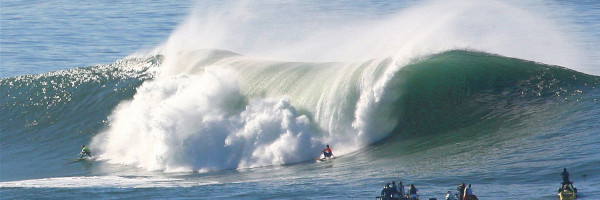By Steve Jurvetson from Menlo Park, USA - Step Into Liquid, CC BY 2.0, link

# Mixing Waves

This is starting to look much better, but it still looks very plain. Real wave crests are never quite quite the same size, and their spacing is irregular too. Does that mean you need to use random numbers? Nope! Real water waves are almost always a composite of many simpler waves just like ours above. Individually they move in extremely predictable ways, but when mixed together they gain a random quality.

Water waves have a trick that helps with this random appearance too. Unlike sound or light, water waves don’t all move at the same speed. (Think about how weird that is for a second…) Specifically, a wave’s speed is proportional to the square root of it’s wavelength, so we just need to multiply that into our time factor like this:

``````y = amplitude*cos(x/wavelength - time*sqrt(wavelength)/wavelength)
``````

Which simplifies to:

``````y = amplitude*cos(x/wavelength - time/sqrt(wavelength))
``````

If we plot these waves separately, you can see that the blue wave has 4x the wavelength of the red wave, and moves 2x as fast. At least to my eyes, this just doesn’t look right when you see them side by side.

Mixing waves is easy, you just add them together. Trochoidal waves have a vertical and horizontal component, so you just add them all up separately. (x, y, and z if doing 3D) Once mixed, we get the signature “pulsing” look of real waves.

Edit this code!

• Try different wavelengths
• What happens if you pick wavelengths that are similar?
• Can you cause the wave to break by adding smaller waves that are fine independently?
• Try dividing time by wavelength like before instead of it’s square root
• Try dividing time by a constant

If Mixing two waves looks so nice, you’d be correct to think that mixing more waves can look even better. The problem is how many do you need? For each additional wave you need to calculate a whole lot more sines and cosines. It gets even worse when you need to do this in 3D. CPUs and GPUs are insanely fast, but at some point brute forcing a problem won’t work. Also, wasn’t this supposed to be an article about interactive water simulation? How on earth do you interact with sine waves!?

## Mix ALL the Waves!

Since you already know that this article is about using the FFT to simulate water, perhaps you won’t be surprised when I reveal that the FFT is the magical solution that can calculate 10’s of thousands of sines and cosines without the performance cost. Even better, you don’t really even need to know much about trigonometry to use it. If you followed along with the scrolling sine waves above, you should be able to understand the rest of the article just fine.

# Complex Numbers

The FFT operates on complex numbers, so we should talk about the basics. Don’t let the name fool you though, they’re just like 2D vectors with many common properties. Addition works the same, and calculating their length or direction works the same way. Instead of x and y coordinates, they have “real” and “imaginary” parts that mean the same thing. When we work with the FFT to make a wave, it will give us the wavelength and we tell it the amplitude and phase shift of the wave using the length and the angle of a complex number we give back. It works like this:

The length of a complex number sets the amplitude of the wave, and the angle sets it’s phase.

The reason why the FFT uses complex numbers is because they are a shortcut for a bunch of trigonometry stuff. All you really need to know for this article is that multiplying two complex numbers adds their angles and multiplies their lengths. In other words, it does rotation and scaling in one step. The underlying math ends up being just a few regular additions and multiplications which is great for performance. Magic!

There’s a lot more neat stuff to discover with complex numbers, like fractals or why they use real and imaginary instead of x and y, but that’s all you need to know for now.

# The FFT Algorithm

The most intuitive way to think of the Fast Fourier Transform, or FFT, is a way to break a sequence of numbers into an equivalent list of waves. If we ran our mixed wave from the last example through the FFT, it would separate it back into the red and blue waves. While you can add these waves back together yourself, it gets expensive very quickly since you have to compute a new list of sines and cosines for each wave. Instead you can undo the FFT, and mix those waves back together using the inverse FFT. If you were mixing them yourself, each additional wave would cost the same amount of performance, but when using the FFT each additional wave costs less than the one before it. Even better, because it uses complex number tricks, the FFT can skip nearly every single call to (mildly) expensive functions like `sin()` and `cos()`!

Time to get our feet wet! The 16 pairs of sliders on the left are the height and velocity at each grid point of the water. They come in pairs because they are actually complex numbers: height = real, velocity = imaginary. The 16 pairs on the right side are the amplitude and phase of the waves the water decomposes into. These are also complex numbers, but drawn using the length and angle since those are the wave properties we are interested in. Right away, you can see when the water surface looks like a sine wave, it decomposes into a single wave.

Use the left mouse button to change values, or right mouse button to clear them.

• What does the first wave value represent?
• What’s the difference between the 2nd and last waves?
• How does the phase affect the 2nd and last wave differently?
• Which waves have long or short wavelengths?
• What’s different about the 9th wave?
• Does changing a single water value change all waves?
• Does changing a single wave value change all of the water?
• The height and velocity always look similar. Do you remember why?

Now for some boring FFT details we need to know: When given a grid of N water surface points, the FFT will split it into N waves, and N must be a power of two. (like 16, 256, 1024, etc) It also treats the input as a repeating sequence. This is extremely handy if you want to make the results tileable, but mildly annoying if you don’t. To avoid wrapping around you’ll just need to save some space at the edges to clamp the height/velocity values to 0.

The wavelengths the FFT uses always follow a simple pattern: The first wave is just the average water height/velocity (think wavelength = infinity). Then next few waves will have wavelengths N/1, N/2, N/3 and so on. Eventually when you get to the middle, you’ll have a wavelength of N/(N/2), which simplifies to 2. Now the waves switch directions, and the wavelengths start getting longer until you get to the final wave with a wavelength of N/1 again. Put another way, the waves have wavelength N/index in the first half and N/(N - index) in the second half.

# Using the FFT on a 2D Grid for a 3D Game

To use the FFT for 3D water, you would apply it to the rows of the grid first, then apply it to the columns. (the order doesn’t actually matter) It might seem weird to apply the FFT to the columns when they already contain wave information, but it works! The FFT is a separable filter so you can take this nice computational shortcut. There’s a few extra details you need to know for water in a 3D game, but there are plenty of resources for that once you know these basics.

# Animating Water with the FFT

With all that out of the way, let’s animate some water with 64 waves and see what it looks like. Remember we have 3 wave parameters: wavelength, amplitude, and speed. We can pick the amplitudes ahead of time using the grey slider bars, the wavelenths define the speed `-time/sqrt(wavelength)`, and the inverse FFT defines the wavelengths we will use. Let’s simplify things even further for now by ignoring the backwards moving waves (the second half of them), and since N is a constant for all waves we can just use 1/i as the wavelength expression when calculating the speed. We can multiply the whole thing by a constant instead to control the time scale of all the waves leaving us with just `-time*time_scale*sqrt(i)` for the wave speed. The last little detail is that the grey bars will give us a complex number with a length controlled by the bar, and a random starting phase. (Waves kind of explode if their phases all line up at the same time, and that doesn’t really happen in nature.) All we need to do then is add our animated phase angle to the wave’s complex number while keeping it’s length. Remember that complex multiplication lets us add angles and multiply lengths. So if we multiply by `complex(cos(angle), sin(angle)`, then we get our angle with a length of 1.

Edit this code!

• Experiment with the wave speeds.
• Can you make the waves all move the same speed?
• Can you make the waves move to the left?
• What happens if you negate the sin() or cos()? Do you know why?
• What happens if you replace the sin() or cos() with zero?
• Does the animation work if you add backwards waves? (The bars on the right half)

This is looking pretty good. The peak heights and spacing are all different and always changing. It looks random yet it’s entirely predictable from the initial state set by the gray bars. We can easily predict what the water will look like at any time in the future or past with just 5 lines of code! To draw it, the “real” parts of the numbers are the sum of the cosines, and the “imaginary” parts are the sum of the cosines. These substite right into the old drawing code you wrote before like this:

``````x_out[i] = i - water[i].imaginary;
y_out[i] = water[i].real
``````

Now, since we ignored handling the wavelengths of the backwards waves (the ones in the second half), so it’s not suprising that they make things glitchy. That’s easy enough to fix though, we can just process the waves in forwards/backwards pairs that share a wavelength. The other issue is that we are sort of abusing the water’s imaginary number component. It’s supposed to be the velocity of the water’s surface, and it’s only a coincedence that it’s the same value we needed for the trochoidal motion. For backwards moving waves it goes negative and turns the trochoidal shape upside down. Drat! The fix for that is a bit more annoying as we need to make a second set of waves for the x motion. The forward moving waves need to be 90° behind, and the backwards moving waves 90° ahead. We can just swap some coordinates to make the right angle changes. Now we have two arrays of complex numbers for the x and y positions of the water, and use the “real” values of each to draw it.

Edit this code!

• Try adding both forwards and backwards moving waves.
• Try experimenting with the `waves_x` values to see how it changes the wave shapes.

This is quite a bit more complicated than the last code snippet, but now we can animate basically any sort of surface wave interaction.

## Water Simulation

This article promised water simulation, but everything has been only about animation so far. Don’t ask for your money back just yet! We only need a few extra changes to turn our animation into a full blown simulation. It’s easy to understand how to interact with the water as a grid. You change the position or velocity of certain cells to match objects interacting with the surface. It’s not obvious how to interact with a list of waves though. The great part about working with fourier transforms is that you can use both representations and switch between them quickly. We’ve only been using the inverse FFT to switch in one direction, but we can go both ways.

We’ll keep the water interaction simple. If the mouse is down, push down the water height of the cells near the mouse. Then we convert the water surface to waves using the FFT and animate them similar to before, but with a couple differences. First, since we aren’t animating the waves from an initial state, we need to change the time to advance from the previous frame instead (delta_time). Second, to make the waves lose energy over time, we apply some damping by multiplying the phase by an exponential term to make it’s length a little less than 1.0. Shorter waves lose energy faster, so I multiplied that in too. It looks ok, but I have no idea if that particular math is physically accurate.

Edit this code!

• Can you make a better interaction than pushing the height down in a square?
• I set the first wave to 0. What happens if you remove that? Do you know why?
• Try clicking and dragging the mouse to make a big wave.

The code snippet has gotten pretty long at this point, but it didn’t take much more to turn our animation into a simulation. The last problem to solve is that there is nothing to stop breaking waves from happening. Before we were animating waves that were in equilibrium, as if they were blown along by the wind perhaps. We could simply hand tweak the wave amplitudes to prevent breaking waves. Now the waves are set by the simulation state. The easiest solution is to scale down the x component of the trochoidal motion, or remove it altogether. Otherwise we’ll have to detect breaking crests where they happen. Scan through the water grid and look at the x values to see if the mesh vertexes will move beyond their neighbors. If they do, then scale down their height and velocity to decrease the energy at their location. You could use this information to add splashes or foam to the wave crest when drawing too I suppose. The code for that looks like the following. You can paste it into the last example after the `if(click_x){}` block if you want to try it out.

``````let n = water_y.n;
for(let i = 0; i < n; i++){
let x_prev = water_x[(i - 1 + n)%n].re;
let x_curr = water_x[i].re;
let x_next = water_x[(i + 1)%n].re;
let xdiff_max = max(x_curr - x_next, x_prev - x_curr);
if(xdiff_max > 1){
let coef = complex(1/xdiff_max, 0);
water_y[i] = complex_multiply(water_y[i], coef);
}
}
``````

## Closing thoughts:

Hopefully this article has given you a bit of intuition for the FFT, and a better understanding of the underlying math of waves. I put a lot of hours into making all the interactive content for this article, but I’m a complete Javascript dunderhead. If you know how to improve on any of this please let me know!

The keen eyed DSP die hards among you might have noticed that updating the waves is a convolution. The filter impulse is the same length as the FFT window, so it wouldn’t be practical for an animation, but for a small time step you could approximate it with a truncated FIR filter. This would definitely work, and I’d be interested to try it out someday to see if the accuracy and performance tradeoffs are worthwhile.

If you made it to the end, then I’m sorry for this, but it simply had to be done: Thanks for taking the plunge with me as we dove into this algorithm. Now it’s time for one final wave… goodbye.